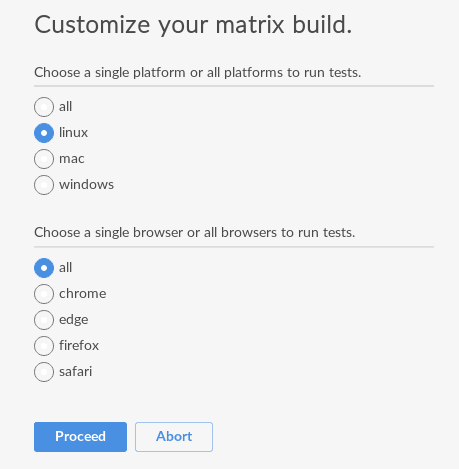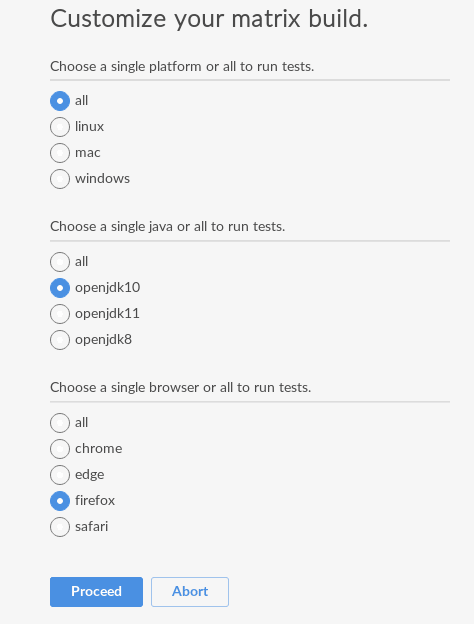# Matrix building in scripted pipeline

With the recent announcement about matrix building you can perform Matrix builds with declarative pipeline. However, if you must use scripted pipeline, then I’m going to cover how to matrix build platforms and tools using scripted pipeline. The examples in this post are modeled after the declarative pipeline matrix examples.

## Matrix building with scripted pipeline

The following Jenkins scripted pipeline will build combinations across two matrix axes. However, adding more axes to the matrix is just as easy as adding another entry to the `Map matrix_axes`.

Jenkinsfile
``````// you can add more axes and this will still work
Map matrix_axes = [
PLATFORM: ['linux', 'windows', 'mac'],
BROWSER: ['firefox', 'chrome', 'safari', 'edge']
]

@NonCPS
List getMatrixAxes(Map matrix_axes) {
List axes = []
matrix_axes.each { axis, values ->
List axisList = []
values.each { value ->
axisList << [(axis): value]
}
axes << axisList
}
// calculate cartesian product
axes.combinations()*.sum()
}

// filter the matrix axes since
// Safari is not available on Linux and
// Edge is only available on Windows
List axes = getMatrixAxes(matrix_axes).findAll { axis ->
!(axis['BROWSER'] == 'safari' && axis['PLATFORM'] == 'linux') &&
!(axis['BROWSER'] == 'edge' && axis['PLATFORM'] != 'windows')
}

for(int i = 0; i < axes.size(); i++) {
// convert the Axis into valid values for withEnv step
Map axis = axes[i]
List axisEnv = axis.collect { k, v ->
"\${k}=\${v}"
}
// let's say you have diverse agents among Windows, Mac and Linux all of
// which have proper labels for their platform and what browsers are
// available on those agents.
String nodeLabel = "os:\${axis['PLATFORM']} && browser:\${axis['BROWSER']}"
node(nodeLabel) {
withEnv(axisEnv) {
stage("Build") {
echo nodeLabel
sh 'echo Do Build for \${PLATFORM} - \${BROWSER}'
}
stage("Test") {
echo nodeLabel
sh 'echo Do Build for \${PLATFORM} - \${BROWSER}'
}
}
}
}
}

stage("Matrix builds") {
}``````

Matrix axes contain the following combinations:

``````[PLATFORM=linux, BROWSER=firefox]
[PLATFORM=windows, BROWSER=firefox]
[PLATFORM=mac, BROWSER=firefox]
[PLATFORM=linux, BROWSER=chrome]
[PLATFORM=windows, BROWSER=chrome]
[PLATFORM=mac, BROWSER=chrome]
[PLATFORM=windows, BROWSER=safari]
[PLATFORM=mac, BROWSER=safari]
[PLATFORM=windows, BROWSER=edge]``````
 It is worth noting that Jenkins agent labels can contain a colon (`:`). So `os:linux` and `browser:firefox` are both valid agent labels. The node expression `os:linux && browser:firefox` will search for Jenkins agents which have both labels.

## Screenshot of matrix pipeline

The following is a screenshot of the pipeline code above running in a sandbox Jenkins environment.It is useful for users to be able to customize building matrices when a build is triggered. Adding static choices requires only a few changes to the above script. Static choices as in we hard code the question and matrix filters.

Jenkinsfile
``````Map response = [:]
stage("Choose combinations") {
response = input(
id: 'Platform',
parameters: [
choice(
choices: ['all', 'linux', 'mac', 'windows'],
description: 'Choose a single platform or all platforms to run tests.',
name: 'PLATFORM'),
choice(
choices: ['all', 'chrome', 'edge', 'firefox', 'safari'],
description: 'Choose a single browser or all browsers to run tests.',
name: 'BROWSER')
])
}

// filter the matrix axes since
// Safari is not available on Linux and
// Edge is only available on Windows
List axes = getMatrixAxes(matrix_axes).findAll { axis ->
(response['PLATFORM'] == 'all' || response['PLATFORM'] == axis['PLATFORM']) &&
(response['BROWSER'] == 'all' || response['BROWSER'] == axis['BROWSER']) &&
!(axis['BROWSER'] == 'safari' && axis['PLATFORM'] == 'linux') &&
!(axis['BROWSER'] == 'edge' && axis['PLATFORM'] != 'windows')
}``````

The pipeline code then renders the following choice dialog.When a user chooses the customized options, the pipeline reacts to the requested options.Dynamic choices means the choice dialog for users to customize the build is generated from the `Map matrix_axes` rather than being something a pipeline developer hard codes.

For user experience (UX), you’ll want your choices to automatically reflect the matrix axis options you have available. For example, let’s say you want to add a new dimension for Java to the matrix.

``````// you can add more axes and this will still work
Map matrix_axes = [
PLATFORM: ['linux', 'windows', 'mac'],
JAVA: ['openjdk8', 'openjdk10', 'openjdk11'],
BROWSER: ['firefox', 'chrome', 'safari', 'edge']
]``````

To support dynamic choices, your choice and matrix axis filter needs to be updated to the following.

``````Map response = [:]
stage("Choose combinations") {
response = input(
id: 'Platform',
parameters: matrix_axes.collect { key, options ->
choice(
choices: ['all'] + options.sort(),
description: "Choose a single \${key.toLowerCase()} or all to run tests.",
name: key)
})
}

// filter the matrix axes since
// Safari is not available on Linux and
// Edge is only available on Windows
List axes = getMatrixAxes(matrix_axes).findAll { axis ->
response.every { key, choice ->
choice == 'all' || choice == axis[key]
} &&
!(axis['BROWSER'] == 'safari' && axis['PLATFORM'] == 'linux') &&
!(axis['BROWSER'] == 'edge' && axis['PLATFORM'] != 'windows')
}``````

It will dynamically generate choices based on available matrix axes and will automatically filter if users customize it. Here’s an example dialog and rendered choice when the pipeline executes.## Full pipeline example with dynamic choices

The following script is the full pipeline example which contains dynamic choices.

``````// you can add more axes and this will still work
Map matrix_axes = [
PLATFORM: ['linux', 'windows', 'mac'],
JAVA: ['openjdk8', 'openjdk10', 'openjdk11'],
BROWSER: ['firefox', 'chrome', 'safari', 'edge']
]

@NonCPS
List getMatrixAxes(Map matrix_axes) {
List axes = []
matrix_axes.each { axis, values ->
List axisList = []
values.each { value ->
axisList << [(axis): value]
}
axes << axisList
}
// calculate cartesian product
axes.combinations()*.sum()
}

Map response = [:]
stage("Choose combinations") {
response = input(
id: 'Platform',
parameters: matrix_axes.collect { key, options ->
choice(
choices: ['all'] + options.sort(),
description: "Choose a single \${key.toLowerCase()} or all to run tests.",
name: key)
})
}

// filter the matrix axes since
// Safari is not available on Linux and
// Edge is only available on Windows
List axes = getMatrixAxes(matrix_axes).findAll { axis ->
response.every { key, choice ->
choice == 'all' || choice == axis[key]
} &&
!(axis['BROWSER'] == 'safari' && axis['PLATFORM'] == 'linux') &&
!(axis['BROWSER'] == 'edge' && axis['PLATFORM'] != 'windows')
}

for(int i = 0; i < axes.size(); i++) {
// convert the Axis into valid values for withEnv step
Map axis = axes[i]
List axisEnv = axis.collect { k, v ->
"\${k}=\${v}"
}
// let's say you have diverse agents among Windows, Mac and Linux all of
// which have proper labels for their platform and what browsers are
// available on those agents.
String nodeLabel = "os:\${axis['PLATFORM']} && browser:\${axis['BROWSER']}"
node(nodeLabel) {
withEnv(axisEnv) {
stage("Build") {
echo nodeLabel
sh 'echo Do Build for \${PLATFORM} - \${BROWSER}'
}
stage("Test") {
echo nodeLabel
sh 'echo Do Build for \${PLATFORM} - \${BROWSER}'
}
}
}
}
}

stage("Matrix builds") {
}``````

## Background: How does it work?

The trick is in `axes.combinations()*.sum()`. Groovy combinations are a quick and easy way to perform a cartesian product.

Here’s a simpler example of how cartesian product works. Take two simple lists and create combinations.

``````List a = ['a', 'b', 'c']
List b = [1, 2, 3]

[a, b].combinations()``````

The result of `[a, b].combinations()` is the following.

``````[
['a', 1],
['b', 1],
['c', 1],
['a', 2],
['b', 2],
['c', 2],
['a', 3],
['b', 3],
['c', 3]
]``````

Instead of `a, b, c` and `1, 2, 3` let’s do the same example again but instead using matrix maps.

``````List java = [[java: 8], [java: 10]]
List os = [[os: 'linux'], [os: 'freebsd']]

[java, os].combinations()``````

The result of `[java, os].combinations()` is the following.

``````[
[ [java:8],  [os:linux]   ],
[ [java:10], [os:linux]   ],
[ [java:8],  [os:freebsd] ],
[ [java:10], [os:freebsd] ]
]``````

In order for us to easily use this as a single map we must add the maps together to create a single map. For example, adding `[java: 8] + [os: 'linux']` will render a single hashmap `[java: 8, os: 'linux']`. This means we need our list of lists of maps to become a simple list of maps so that we can use them effectively in pipelines.

To accomplish this we make use of the Groovy spread operator (`*.` in `axes.combinations()*.sum()`).

Let’s see the same `java`/`os` example again but with the spread operator being used.

``````List java = [[java: 8], [java: 10]]
List os = [[os: 'linux'], [os: 'freebsd']]

[java, os].combinations()*.sum()``````

The result is the following.

``````[
[ java: 8,  os: 'linux'],
[ java: 10, os: 'linux'],
[ java: 8,  os: 'freebsd'],
[ java: 10, os: 'freebsd']
]``````

With the spread operator the end result of a list of maps which we can effectively use as matrix axes. It also allows us to do neat matrix filtering with the `findAll {}` Groovy `List` method.

## Exposing a shared library pipeline step

The best user experience is to expose the above code as a shared library pipeline step. As an example, I have added `vars/getMatrixAxes.groovy` to Jervis. This provides a flexible shared library step which you can copy into your own shared pipeline libraries.

The step becomes easy to use in the following way with a simple one dimension matrix.

Jenkinsfile
``````Map matrix_axes = [
PLATFORM: ['linux', 'windows', 'mac'],
]

List axes = getMatrixAxes(matrix_axes)

// alternately with a user prompt
//List axes = getMatrixAxes(matrix_axes, user_prompt: true)``````

Here’s a more complex example using a two dimensional matrix with filtering.

Jenkinsfile
``````Map matrix_axes = [
PLATFORM: ['linux', 'windows', 'mac'],
BROWSER: ['firefox', 'chrome', 'safari', 'edge']
]

List axes = getMatrixAxes(matrix_axes) { Map axis ->
!(axis['BROWSER'] == 'safari' && axis['PLATFORM'] == 'linux') &&
!(axis['BROWSER'] == 'edge' && axis['PLATFORM'] != 'windows')
}``````

And again with a three dimensional matrix with filtering and prompting for user input.

Jenkinsfile
``````Map matrix_axes = [
PLATFORM: ['linux', 'windows', 'mac'],
JAVA: ['openjdk8', 'openjdk10', 'openjdk11'],
BROWSER: ['firefox', 'chrome', 'safari', 'edge']
]

List axes = getMatrixAxes(matrix_axes, user_prompt: true) { Map axis ->
!(axis['BROWSER'] == 'safari' && axis['PLATFORM'] == 'linux') &&
!(axis['BROWSER'] == 'edge' && axis['PLATFORM'] != 'windows')
}``````

The script approval is not necessary for Shared Libraries.

If you don’t want to provide a shared step. In order to expose matrix building to end-users, you must allow the following method approval in the script approval configuration.

Script approval
``staticMethod org.codehaus.groovy.runtime.DefaultGroovyMethods combinations java.util.Collection``

## Summary

We covered how to perform matrix builds using scripted pipeline as well as how to prompt users for customizing the matrix build. Additionally, an example was provided where we exposed getting buildable matrix axes to users as an easy to use Shared Library step via `vars/getMatrixAxes.groovy`. Using a shared library step is definitely the recommended way for admins to support users rather than trying to whitelist groovy methods.

Jervis shared pipeline library has supported matrix building since 2017 in Jenkins scripted pipelines. (see here and here for an example).